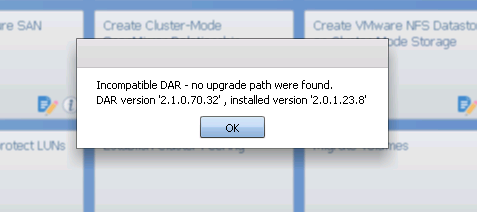Data Infrastructure Management Software Discussions

## Function to calculate new volume size - problemI am attempting to create a function that calculates correct volume size based on a desired percentage used. I am encountering what I believe to be a computational issue inside the MVEL where it doesn't behave consistently. Maybe this is a bug, or maybe the issue is between my ears? I need some help, though.

Here is the code:

def calculateTargetVolumeSize(vol_used,vol_avail,snap_percent,snap_used,target_percent)

{

int percent_used = (vol_used / (vol_used + vol_avail)) * 100;

if (percent_used < target_percent ) {

throwException("Percent used " + percent_used + "% is already below designated percentage of " + target_percent + "%.\nThe following values were passed: " + vol_used + ", " + vol_avail + ", " + snap_percent + ", " + snap_used);

}

int new_avail_size = (int)(vol_used / (target_percent / 100));

int new_vol_size = (int)(new_avail_size / (1 - (snap_percent / 100)));

int new_snap_size = new_vol_size - new_avail_size;

if (snap_used > new_snap_size) {

new_vol_size = new_avail_size + snap_used;

}

if (new_vol_size < 20 ) {

new_vol_size = 20;

}

// throwException("new size: " + new_vol_size);

return new_vol_size;

}

I am leveraging this function in a workflow that uses the Resize volume command. The function is in the TargetSize value. It returns the following error:

Function: 'calculateTargetVolumeSize'

With parameters: v1.used_size_mb, v1.available_size_mb, v1.snapshot_reserved_percent, v1.snapshot_used_mb, 70

Threw an Exception with a message: Percent used 9.99997731789257E-4% is already below designated percentage of 70%.

The following values were passed: 237883, 53957, 5, 50035

I cannot for the life of me figure out how ( 237883 / (237883 + 53957)) * 100 = 9.999977331789257E-4

When I run the function as a test using this syntax: calculateTargetVolumeSize(237883,53957,5,50035,70)

It returns the following value correctly: 389867

Any ideas?

Thanks,

Will

9 REPLIES 9

## Re: Function to calculate new volume size - problemWill,

Looking at the function, I can't make out anything wrong, it seems fine. Can you post the workflow where you are calling the function, it probably is where the problem might be.

If this post resolved your issue, help others by selecting ACCEPT AS SOLUTION or adding a KUDO.

## Re: Function to calculate new volume size - problemThanks for looking into this. Attached is the full workflow.

## Re: Function to calculate new volume size - problemWill,

Is V1 volume variable defined by you or found using a finder?

Just asking to confirm that those attributes of V1 have values. I suspect one of them is not getting the right value.

Can you add all those parameters that you pass to the functions to be return parameters so you can see them in the planning?
You might need to add them and disable the function briefly (Just exchange it with some fixed size) to get through planning and see those values.

Very useful for debugging...

Best,

Yaron

## Re: Function to calculate new volume size - problemThanks Yaron,

I did as you recommended and the values returned are in the attached screenshot.

Thanks,

Will

## Re: Function to calculate new volume size - problemhey willwalsh,

I would like to know which version of WFA you have installed. is it 1.0 ?

Sinhaa,

I know this is completely off track from what we are looking at here but, I get an error while importing this workflow given by willwalsh in my setup. I have attached the snapshot of the error.I have WFA 2.0.1.23.8 installed. I saw another thread (https://communities.netapp.com/thread/25651) on importing workflows from 1.0 to 2.0 and the outcome of the thread says that we need an intermediary installation of 1.1.1 to get it done. Is there no other way of doing it ?

## Re: Function to calculate new volume size - problemI am running version 2.1.0.70.32

## Re: Function to calculate new volume size - problemI realize that I did not answer part of your question. v1 is found using a finder. I ask the user to input the volume in the format <filer>:/vol/<volume>. I then parse that into the variables filer and volume and pass that to the finder. The values appear to be correct, both as returned values, and when the function throws an exception.

## Re: Function to calculate new volume size - problemHey,

You are running into precision issues with the math. As the input is integer, it is being converted along the way and integers cannot hold decimal places. Hence you get enormous rounding errors. ie. 1/3 = 0.333 as a double, or 0 as an integer.

Try something like this instead, force type-cast as double the variables for the calculations:

int percent_used = (((double)vol_used) / (((double)vol_used) + ((double)vol_avail))) * 100;

This will result in the correct response as the 'double' type can store many decimal places during the calculation. Keep in mind casting is a low precedence operator on MVEL unlike some other languages and needs the brackets. Without them, the first divide would be interpreted with vol_used as an integer instead then converted to double after the divide, but it's already lost the decimal places at this point. It's safest to always use brackets in MVEL when type-converting, and remember that MVEL will automatically type-convert for you which may have unexpected side effects like precision loss.

Regards,

Michael.

## Re: Function to calculate new volume size - problemThanks Michael! That fixed it. Here is the completed workflow. For those interested in using it. It was created in WFA 2.1.

Thanks,
Will